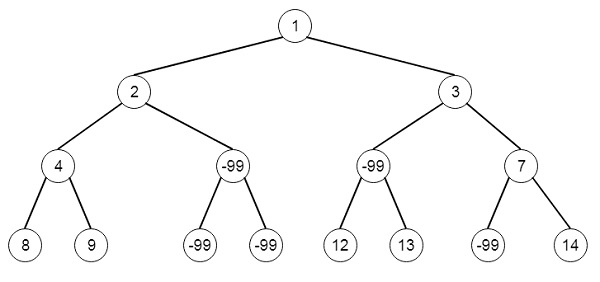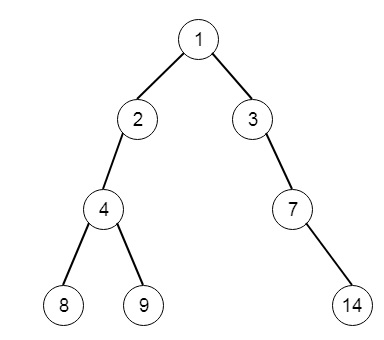# Insufficient Nodes in Root to Leaf Paths in Python

PythonServer Side ProgrammingProgramming

Suppose we have a binary tree. A node is known as insufficient if every such root to leaf path intersecting this node has sum strictly less than limit. We have to delete all insufficient nodes simultaneously, and return the root of the resulting binary tree. So if the tree is like, and the limit is 1 −Then the output tree will be −To solve this, we will follow these steps −

• Define a method solve(), this will take root and limit
• if node has no left and right subtree, then
• if value of root is less than 1, return null, otherwise root
• if root has left subtree, then left of root := solve(left of root, limit – value of root)
• if root has right subtree, then right of root := solve(right of root, limit – value of root)
• if root has either left subtree, or right subtree or both, then return root, otherwise null.

Let us see the following implementation to get better understanding −

## Example

class Solution(object):
def sufficientSubset(self, root, limit):
"""
:type root: TreeNode
:type limit: int
:rtype: TreeNode
"""
if not root.left and not root.right:
return None if root.val<limit else root
if root.left:
root.left= self.sufficientSubset(root.left,limit-root.val)
if root.right:
root.right = self.sufficientSubset(root.right,limit-root.val)
return root if root.left or root.right else None

## Input

[1,2,3,4,-99,-99,7,8,9,-99,-99,12,13,-99,14]
1

## Output

[1,2,3,4,null,null,7,8,9,null,14]